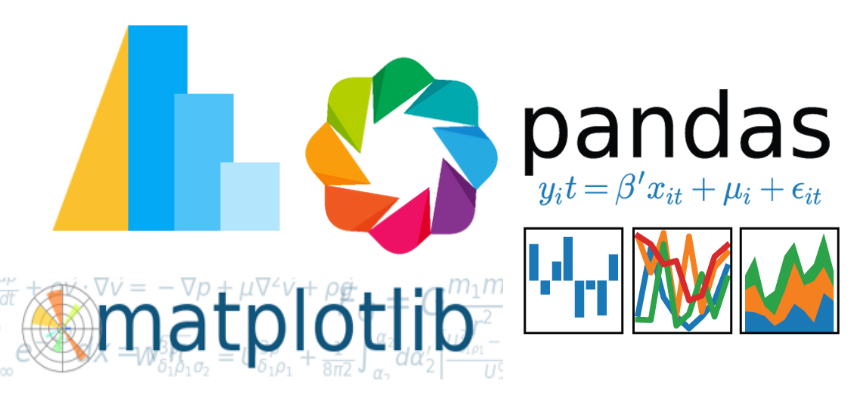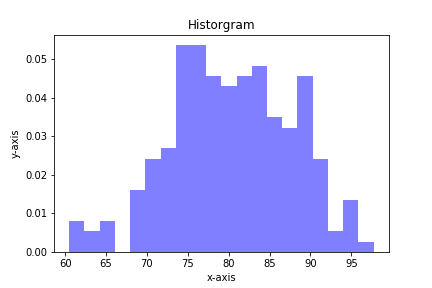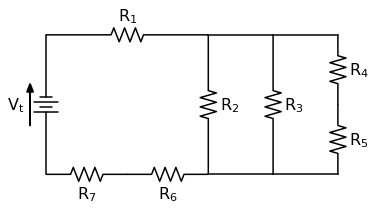## Calculate the Probability Under a Normal CurveCalculating the probability under a normal curve is useful for engineers. This type of calculation can be helpful to predict the likely hood of a part coming off an assembly line being within a given specification. The probability can be calculated when the statistical properties of all the parts that …

## Quiver plots using Python, matplotlib and Jupyter notebooksA quiver plot is a type of 2D plot that shows vector lines as arrows. Quiver plots are useful in electrical engineering to visualize electrical potential and valuable in mechanical engineering to show stress gradients. In this post, we will build three quiver plots using Python, matplotlib, numpy, and Jupyter notebooks.

## Plotting a stress-strain curve with four libraries: matplotlib, pandas, altair and bokehAfter watching a great webinar about plotting with different python libraries, I wanted to see what it was like to make a stress strain curve using four different modules: pandas, matplotlib, altair and bokeh (with holoviews).

## Plotting Histograms with matplotlib and PythonHistograms are a useful type of statistics plot for engineers. A histogram is a type of bar plot that shows the frequency or number of values compared to a set of value ranges. Histogram plots can be created with Python and the plotting package matplotlib. The plt.hist() function creates …

## Solving a Circuit Diagram Problem with Python and SchemDraw

In this post we will solve a circuit diagram problem using a Python package called SchemDraw